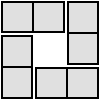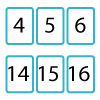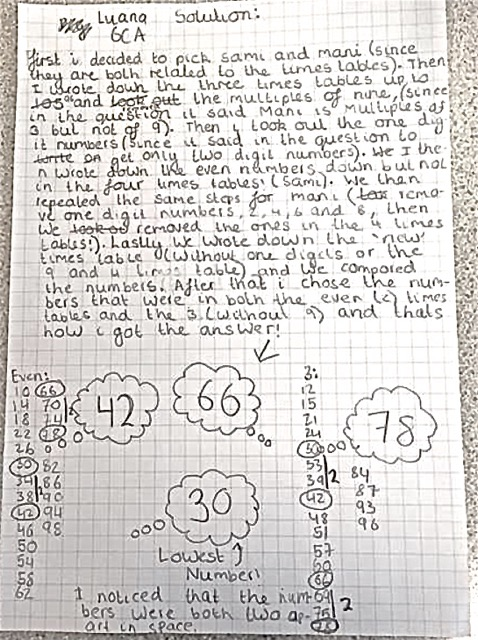#### You may also like### 4 Dom

Use these four dominoes to make a square that has the same number of dots on each side.### Twenty Divided Into Six

Katie had a pack of 20 cards numbered from 1 to 20. She arranged the cards into 6 unequal piles where each pile added to the same total. What was the total and how could this be done?### Mystery Matrix

Can you fill in this table square? The numbers 2 -12 were used to generate it with just one number used twice.

# Satisfying Four Statements

##### Age 7 to 11Challenge Level
The first edition of this challenge referred to Alison, Becky, Sam and Matt and these two solutions came in.

Aryaman from Bangkok Patana School in Thailand sent in this very good solution:

Alison (Multiples of 5): {5,10,15,20,25,...}
Becky (Triangular numbers): {1,3,6,10,15,21,...}
Sam (Even but not multiples of 4): {2,6,10,14,18,22,...}
Matt (Multiples of 3 but not multiples of 9): {3,6,12,15,21,24,...}
Some of the the 2 digit numbers that belong in two of the sets are:
21 belongs in the sets of both Matt and Becky. 10 also belongs in the sets of Alison and Becky.
Some of the the 2 digit numbers that belong in three of the sets are:
15 belongs in the sets of Alison, Becky and Matt.
To find the smallest numbers that belongs in all 4 sets I first found the list of the first 100 triangular numbers. I also realised it has to end with 0 because it has to be both divisible by 5 and even. Therefore from the list of triangular numbers I took the numbers that end with 0 and check whether they are multiples of 4 and divisible by 3 and 9. For example, I had to exclude 120 as it is divisible by 4. I also excluded 190 as it is not divisible by 3. The number that met all the requirements was 210:
1. It is divisible by 5 and even but not a multiple of 4.
2. It is triangular (as it was on my list of triangular numbers).
3. It is divisible by 3 but not by 9.

List the numbers that satisfy both Alison's and Sam's statements:
{10,30,50,70,90,110,130,150,...}
One way to describe it would be: start at 10 and go up by 20.
Another would be to list odd numbers and put a '0' to at the end of each. For example:
1,3,5,7,9,... but we put a '0' at the end: 10, 30, 50, 70, 90, 110,...
List the numbers that satisfy both Alison's and Matt's statements:
{15, 30, 60, 75, 105, 120, 150, 165}

JunHa, Ezra and Ilman from American Embassy School New Delhi send in a pdf with their work they found answers as follows:

1.Find numbers that belong in two sets
2.Find numbers that belong in three sets
3.Find the smallest numbers that belongs in all 4 sets So
Q 1. 15,21,66,78,10,30,50,70,90,28,42,48,55,60,75 Numbers that meet Alison's and Becky's conditions are 10,15,45,55
Numbers that meet Becky's and Sam's conditions are 10,66,78
Numbers that meet Sam's and Matt's criteria are 30,42,66
A .numbers that are multiples of five as well ase triangular numbers are 10, 15, 45 and 55. Out of these four numbers 10 is the only even no. but it is not the multiple of 3. We did not find any two digit nos that meet all four conditions.
Numbers that have three criteria are 15,66,78,10,30
15 does not match Sam's criteria
66 and 78 are not multiples of 5
10 is not multiple of 3
And 30 is not a triangular numbers.
Their pdf which shows the diagrams they used can be seen here Satisfying Statement .pdf

The second edition of the challenge just used different names and these are the solutions that then came in.

Jemima and Alice from Ballard School

Ash and Sami share the numbers 10, 30, 50, 70, 90 These are multiples of 10 but not multiples of 20.
Ash and Mani share the number 15, 30, 60 and 75. These are multiples of 15 but not multiples of 9.
Sami and Mani share the numbers 6, 30, 42, 66, 78.
Si and Ash share the numbers 10, 15, 45, 55
Si and Mani share the numbers 15, 21, 78
Si and Sami share the numbers 10 and 78
Numbers that meet Matt's and Alison's are 15,30,60,75,
Q 2. 15,66,78,10,30

Regalo and Jesse the Buxton School

First of all, we wrote some of the triangular numbers in a sequence. Then we crossed out the numbers that weren't in the five times tables and the lowest number that remained was ten. Because we wrote the numbers in a sequence we knew that ten was a triangular number, which fits into two of the statements (multiple of 5 and triangular numbers).

To solve the next problem we knew that ten fitted into two of the statements already, as shown above, so we tried to divide it by three and found that it was not divisible. We then decided to write the next number in its triangular sequence, which was 15. 15 also fits into two statements, so we tried to divide it by 3 and it was divisible. Just to be safe, we divided it by 9, but it was not divisible. Therefore, 15 is the number that fits into 3 different statements (Multiply of 5,  riangular number and multiples of 3 but not of 9).

To solve the last problem we wrote out a vast amount of triangular numbers whilst trying to see if they fit into all 4 sets of statements. As we went on, we found some numbers and tried to divide them by 3, 5 and 9. The numbers consisted of 136, 153 etc, we did this because in order for the number to follow all four sets it had to be a multiple of 5, a triangular number, even but not multiples of 4 and multiples of 3 but not 9. We carried on this process until we eventually got to the number 210. It fits into all four statements and was the lowest number that we could find.
After all of this working out, we concluded that 210 was the smallest number that fit into these four statements.

Luana also from the Buxton School sent in a picture of her work.Thank you all for the explanations you've sent in, I am hoping to see more during 2018.